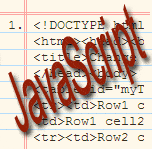Home JAVASCRIPT Apply and arrays Method Using Three tricks For Javascript

## Apply and arrays: three tricks

### The apply method

apply is a method that all functions have. Its signature is```    func.apply(thisValue, [arg1, arg2, ...])
```

Ignoring thisValue, the above invocation is equivalent to:

```    func(arg1, arg2, ...)
```

So, apply lets us unwrap an array “into” the arguments of a function call. Let’s look at three tricks that apply allows us to perform.

### Trick 1: hand an array to a function that does not accept arrays

JavaScript does not have a function that returns the maximum of an array of numbers. However, there is the function Math.max that works with an arbitrary number of number-valued arguments. Thanks to apply, we can use that function for our purposes:

```    > Math.max.apply(null, [10, -1, 5])
10
```

### Trick 2: eliminate holes in arrays

#### Holes in arrays

Quick reminder: In JavaScript, an array is a map from numbers to values. So there is a difference between a missing element (a hole) and an element having the valueundefined . The former is skipped by the Array.prototype iteration methods (forEach, map, etc.), the latter isn’t:

```    > ["a",,"b"].forEach(function (x) { console.log(x) })
a
b

> ["a",undefined,"b"].forEach(function (x) { console.log(x) })
a
undefined
b
```

You can also check for the presence of holes via the in operator.

```    > 1 in ["a",,"b"]
false
> 1 in ["a", undefined, "b"]
true
```

But if you read a hole, the result is undefined for both holes and undefined elements.

```    > ["a",,"b"]
undefined
> ["a", undefined, "b"]
undefined
```

#### Eliminating holes

With apply and Array (which can be used as either a function or a constructor), you can turn holes into undefined elements:

```    > Array.apply(null, ["a",,"b"])
[ 'a', undefined, 'b' ]
```

The above works, because apply does not ignore holes, it passes them as undefinedarguments to the function:

```    > function returnArgs() { return [].slice.call(arguments) }
> returnArgs.apply(null, ["a",,"b"])
[ 'a', undefined, 'b' ]
```

Alas, you are faced with a quirk here. If Array receives a single numeric argument, an empty array is created whose length is the number :

```    > Array.apply(null, [ 3 ])
[ , ,  ]
```

Therefore, if you need this functionality, it is better to write your own function:

```    function fillHoles(arr) {
var result = [];
for(var i=0; i < arr.length; i++) {
result[i] = arr[i];
}
return result;
}
```

Example:

```    > fillHoles(["a",,"b"])
[ 'a', undefined, 'b' ]
```

Underscore gives you the  Compact function which removes all falsy values, including holes:

```    > _.compact(["a",,"b"])
[ 'a', 'b' ]
> _.compact(["a", undefined, "b"])
[ 'a', 'b' ]
> _.compact(["a", false, "b"])
[ 'a', 'b' ]
```

### Trick 3: flatten an array

Task: turn an array of arrays with elements into just an array of elements. Again we use the unwrapping ability of apply to make concat do this work for us:

```    > Array.prototype.concat.apply([], [["a"], ["b"]])
[ 'a', 'b' ]
```

Non-array elements are added as is:

```    > Array.prototype.concat.apply([], [["a"], "b"])
[ 'a', 'b' ]
```

apply’s thisValue must be [] here, because concat is a method, not a non-method function. Only one level of flattening is performed:

```    > Array.prototype.concat.apply([], [[["a"]], ["b"]])
[ [ 'a' ], 'b' ]
```

If you want your code to be self-descriptive, you should consider alternatives, including implementing your own properly named function. Underscore has _.flatten which handles any level of nesting:

`> _.flatten([[["a"]], ["b"]])`
`[ 'a', 'b' ]`
```  ["b"]]
[ 'a', 'b' ]
```
`Non-array elements are added as is:`
```    > Array.prototype.concat.apply([], [["a"], "b"])
[ 'a', 'b' ]
```
`apply’s thisValue must be [] here, because concat is a method, not a non-method function. Only one level of flattening is performed:`
```    > Array.prototype.concat.apply([], [[["a"]], ["b"]])
[ [ 'a' ], 'b' ]
```

If you want your code to be self-descriptive, you should consider alternatives, including implementing your own properly named function. Underscore has _.flatten which handles any level of nesting:

`    > _.flatten([[["a"]], ["b"]])`

#### You may also like